Courses
Courses for Kids
Free study material
Free LIVE classes
More

# NCERT Solutions for Class 7 Maths Chapter 5 - ExerciseLIVE
Join Vedantu’s FREE Mastercalss

## NCERT Solutions for Class 7 Maths Chapter 5 Lines and Angles (EX 5.2) Exercise 5.2

NCERT Solutions for Class 7 Maths Chapter 5 Lines and Angles provided by Vedantu are strictly based on the latest CBSE curriculum. Our solutions to NCERT books for Class 7 Maths come with step by step insight into the chapter. Free PDF downloads of our NCERT Class 7 Maths Chapter 5 Exercise 5.2 has been made available on our learning portal. Subsequently, they can practice these exercises with greater convenience. Download NCERT Solutions PDF for Maths and begin your exam preparation now.  Subjects like Science, Maths, English will become easy to study if you have access to NCERT Solution for Class 7 Science , Maths solutions and solutions of other subjects.

Last updated date: 22nd Sep 2023
Total views: 507.6k
Views today: 11.07k

## Access NCERT Solutions for Class 7 Maths Chapter 5 – Lines And Angles

Exercise 5.2

Refer to pages 7-13 for Exercise 5.2 in the PDF.

1. State the property that is used in each of the following statements?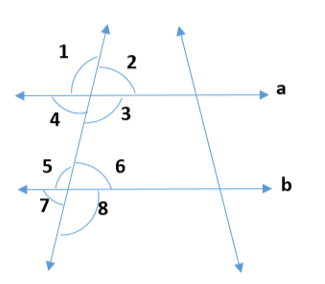i) If \$a\parallel b\$, then \$\angle 1 = \angle 5\$

Ans: Corresponding angles property is used in the above statement.

ii) If \$\angle 4 = \angle 6\$, then \$a\parallel b\$.

Ans : Alternate interior angles property is used in the above statement.

iii) If \$\angle 4 + \angle 5 = 180^\circ \$, then \$a\parallel b\$.

Ans : Interior angles on the same side of transversal are supplementary.

2. In the adjoining figure, identify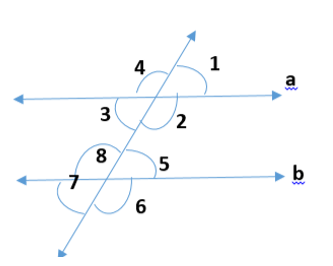i) The pairs of corresponding angles.

Ans : After observing the figure, the pairs of corresponding angles are,

\$\angle 1\$ and \$\angle 5\$, \$\angle 4\$ and \$\angle 8\$, \$\angle 2\$ and \$\angle 6\$, \$\angle 3\$ and \$\angle 7\$.

ii) The pairs of alternate interior angles.

Ans: After observing the figure, the pairs of alternate interior angles are,

\$\angle 2\$ and \$\angle 8\$, \$\angle 3\$ and \$\angle 5\$

iii) The pairs of interior angles on the same side of the transversal.

Ans : After observing the figure, the pairs of interior angles on same side of transversal are,

\$\angle 2\$ and \$\angle 5\$, \$\angle 3\$ and \$\angle 8\$.

iv) The vertically opposite angles.

Ans : After observing the figure, the pairs of vertically opposite angles are,

\$\angle 1\$ and \$\angle 3\$, \$\angle 5\$ and \$\angle 7\$,\$\angle 2\$ and \$\angle 4\$,\$\angle 6\$ and \$\angle 8\$

3. In the adjoining figure, p||q. Find the unknown angle.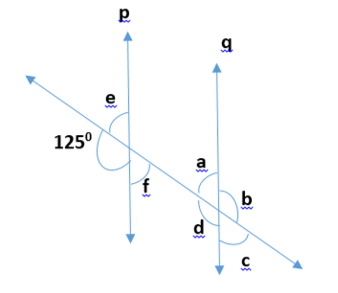Ans: Here,

By observing the figure,

\$\angle d = 125^\circ \$            (corresponding angles)
Also, we know that linear pair is the sum of adjacent angles is 1800

Then,

\$ = \angle e +  + 125^\circ  = 180^\circ \$   (linear pair)

\$ = \angle e = 180^\circ  - 125^\circ \$

\$ = \angle e = 55^\circ \$

From the rule of vertically opposite angles,

\$\angle f = \angle e = 55^\circ \$

\$\angle b = \angle d = 125^\circ \$

By the property of corresponding angles,

\$\angle c = \angle f = 55^\circ \$

\$\angle a = \angle e = 55^\circ \$

4. Find the value of \$x\$in each of the following figures if \$l\parallel m\$.

(I)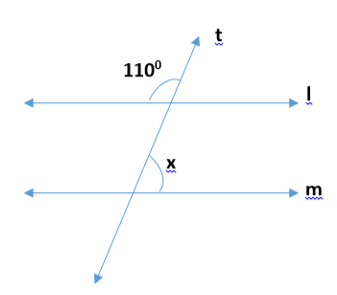Ans : Here, let us assume another angle on the line m be \$\angle y\$.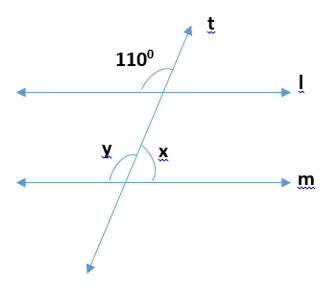Then,

By the property of corresponding angles

\$\angle y = 110^\circ \$

As we know that linear pair is the sum of adjacent angles is \$180^\circ \$

Then,

\$ = \angle x + \angle y = 180^\circ \$

\$ = \angle x + 110^\circ  = 180^\circ \$

\$ = \angle x = 180^\circ  - 110^\circ \$

\$ = \angle x = 70^\circ \$

(ii)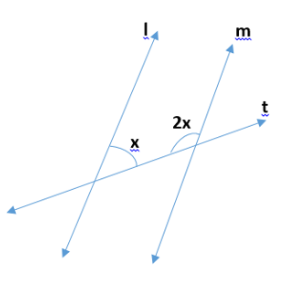Ans: Here,

Given, \$l\parallel m\$ and t is the transversal line.

\$x + 2x = 180^\circ \$                  (Interior opposite angles)

\$ = 3x = 180^\circ \$

\$x = \dfrac{{180^\circ }}{3} = 60^\circ \$

(iii)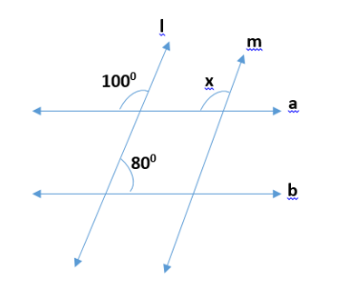Ans : Here,

\$l\parallel m\$ , and \$a\parallel b\$

Therefore,

\$x = 100^\circ \$          (corresponding angles)

5. In the figure, the arms of two angles are parallel.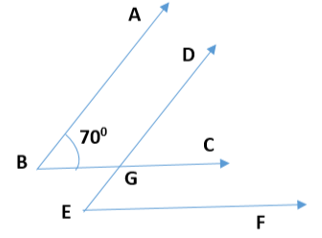If \$\angle ABC = 70^\circ \$, then find

i) \$\angle DGC\$

Ans : Here, let us consider that \$AB\parallel DG\$

By the property of corresponding angles,

\$\angle DGC = \angle ABC\$

Then,

\$\angle DGC = 70^\circ \$

ii) \$\angle DEF\$

Ans: Here, let us consider that \$BC\parallel EF\$

DE is the transversal line intersecting BC and EF

By the property of corresponding angles,

\$\angle DEF = \angle DGC\$

Then,

\$\angle DEF = 70^\circ \$

6. In the figure below, decide whether l is parallel to m.

(I)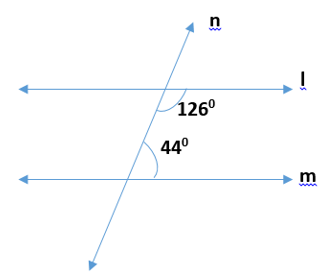Ans: Here, let us consider the two lines \$l\$ and \$m\$.

And n is the transversal line intersecting \$l\$ and \$m\$.

We know that the sum of interior angles on the same side of transversal is \$180^\circ \$

Then,

\$ = 126^\circ  + 44^\circ \$

\$ = 170^\circ \$

But, the sum of interior angles on the same side of transversal is not equal to

\$180^\circ \$.

So, line \$l\$ is not parallel to line \$m\$.

(ii)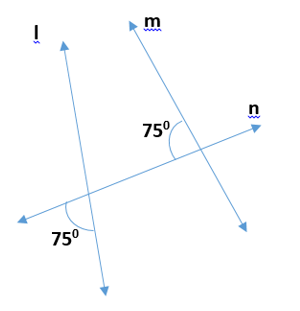Ans: Here, let us assume \$\angle x\$  be the vertically opposite angle formed due to the intersection of the straight line \$l\$ and transversal n,

Then,

\$\angle x = 75^\circ \$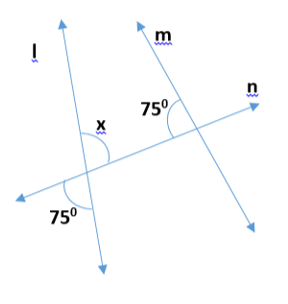Now, let us consider the two lines \$l\$ and \$m\$,

N is the transversal line intersecting \$l\$ and \$m\$.

As we know that the sum of interior angles on the same side of transversal is \$180^\circ \$.

Then,

\$ = 75^\circ  + 75^\circ \$

\$ = 150^\circ \$

But the sum of interior angles on the same side of transversal is not equal to \$180^\circ \$.

So, line \$l\$ is not parallel to line m.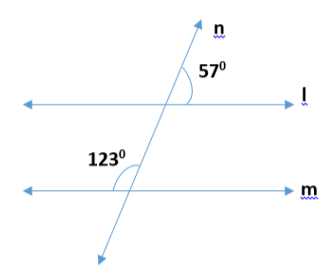(iii)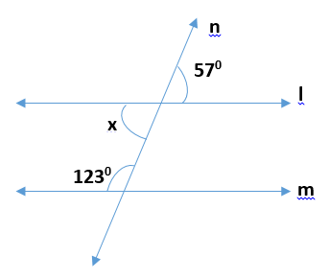Ans: Here, let us assume \$\angle x\$ be the vertically opposite angle formed due to the intersection of the Straight line l and transversal line n,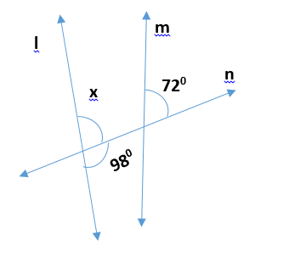Now, let us consider the two lines \$l\$ and m,

N is the transversal line \$l\$ and m.

As we know that the sum of interior angles on the same side of transversal is \$180^\circ \$.

Then,

\$ = 123^\circ  + \angle x\$

\$ = 123^\circ  + 57^\circ \$

\$ = 180^\circ \$

Therefore,  the sum of interior angles on the same side of transversal is equal to \$180^\circ \$

So, line \$l\$ is parallel to line \$m\$.

(iv)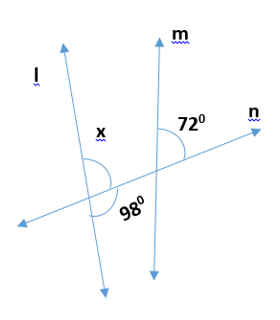Ans: Here, let us assume \$\angle x\$ be the angle formed due to the intersection of the Straight line \$l\$ and transversal line n,

As we know that linear pair is the sum of adjacent angles is equal to \$180^\circ \$.

\$ = \angle x + 98^\circ  = 180^\circ \$

\$ = \angle x = 180^\circ  - 98^\circ \$

\$ = \angle x = 82^\circ \$

Now, as we consider \$\angle x\$ and \$72^\circ \$ are the corresponding angles.

For l and m to be parallel to each other, corresponding angles should be equal.

But, in the given figure corresponding angles measure \$82^\circ \$ and \$72^\circ \$ respectively.

\$\therefore \$ Line \$l\$ is not parallel to line \$m\$.

## Class 7 Maths Chapter 5 Exercise 5.2 – A Summary of Lines and Angles

In this section of the chapter, students get to know the following:

• Refresh their fundamental understanding of lines and angles

• Learn new concepts about its various aspects

For instance, the questions of Class 7 Maths Chapter 5 Exercise 5.2 cover an array of topics and serves as an introduction to concepts such as –

• Properties of parallel lines

• Formation of complementary angles

• Computing angles from given figures

Since most of the problems of Exercise 5.2 are based on these concepts, a detailed understanding of them will come in handy while solving it. In this regard, our Maths NCERT Solutions Class 7 Chapter 5 Exercise 5.2 is deemed the best option. It is because our in-house subject experts with years of experience behind them have drafted our solutions. As a result, the curated solutions are accurate to the ‘T’, cover all the essential pointers and are hence potent enough to fetch excellent scores in exams.

### NCERT Class 7 Maths Solutions Chapter 5 Exercise 5.2 – All Questions

Our solution covers each question of Exercise 5.2 in-depth and offers step by step explanation of each answer. Considering that the provided problems and its patterns are a prevailing see in exams, such explanations are a mandate. It helps you to solve similar problems using our listed out shortcut techniques.

Here’s how our latest Class 7 Maths Chapter 5 Exercise 5.2 Solutions proves beneficial for solving exercise-based questions –

• NCERT Class 7 Maths Chapter 5 Exercise 5.2 – Question 1

Question 1 of Exercise 5.2 consists of 3 parts and requires students to refer to the figure given to answer them accurately. As per the questions, students have been directed to state the specific property of lines and angles that have been used in the given statements. A clear understanding of the features of different lines and angles will come in handy and will allow students to come up with accurate answers.

Consequently, a quick look at our NCERT Class 7 Maths Chapter 5 Exercise 5.2 will help you understand the steps required behind solving these types of problems accurately. Also, remember to look out for similar pattern questions in our offered free-to-download solutions.

• Class 7 Maths Chapter 5 Exercise 5.2 Solutions – Question 2

This said question comprises four subparts and requires students to refer to a given figure to provide accurate answers.

For instance, by taking a close look at the given figure of lines and angles, students have to identify things like - pair of corresponding and alternate interior angles. It also directs students to identify the vertically opposite angles and pairs of interior angles that are said to be on the same side of the transversal.

Figure out the accurate explanations and use of shortcut techniques to solve these types of problems from our NCERT Solution for Class 7 Maths Chapter 5 Exercise 5.2. Doing so, you will be able to solve similar problems with greater ease and will score high in your upcoming exams.

• NCERT Class 7 Maths Chapter 5 Exercise 5.2 – Question 3

In the third question of this exercise, students will learn how to compute the unknown angles from a given figure.

For instance, in this question, students are provided with two parallel lines p and q. With the help of these lines, students have to find out the unknown angles.

In this context, our Class 7 Maths Chapter 5 Exercise 5.2 is deemed the most preferred considering that the answers provided by it, bring in the ease of understanding the chapter. Add on to this the excellent quality of the solutions and that such study guides are not just limited to Maths alone, but are available for all subjects, irrespective of the classes.

• Maths NCERT Solutions Class 7 Chapter 5 Exercise 5.2 – Question 4

This question is accompanied by three pairs of parallel lines named as p and q and tends to test students’ ability to compute the value of x. As per the directions, students have to use the information given and refer to the figure of the parallel lines respectively to solve the problem accurately.

You may refer to our NCERT Maths Class 7 Chapter 5 Exercise 5.2 Solutions if you think you could use a little assistance to solve this type of problem. Additionally, you can also know tricks and tips to solve such problems in a limited time frame.

• Class 7 Maths Chapter 5 Exercise 5.2 Solutions – Question 5

To answer the fifth question of this exercise correctly, students have to refer to the given figure and take account of the information stated carefully.

Learn better techniques of how to find specific angles with limited information by following the solved study materials provided by us and boost your grasp on the chapter further.

• Class 7 Maths Chapter 5 Exercise 5.2 – Question 6

The last question of this exercise tests students’ skill of identifying whether two given lines are parallel to each other or not. By following the figures carefully and applying the fundamentals of lines and angles, one will be able to solve these sorts of problems effortlessly.

To make the problem-solving process further fun and easy, you can also download our latest NCERT Solutions for Class 7 Maths Chapter 5 exercise 5.2. In doing so, you will be able to solve problems with greater convenience.

### Vedantu – Your One-Stop Learning Mate

We, at Vedantu, are a team of qualified teaching professionals adept with years of experience and in-depth knowledge about the scope of the latest CBSE curriculum. Being active in the field of academics helps us come up with study materials that are on par with the CBSE standard and caters to the need of students.

Our exercise-wise study solutions like the NCERT Class 7 Maths Chapter 5 Exercise 5.2 are updated regularly and include step by step explanation for each problem. It helps students understand a variety of problems with ease, and also allow them to develop effective shortcut techniques to solve them with low to no mistakes.

Anybody can access our free PDF download of books and study solutions for free by simply clicking the download option. It is followed by equipping oneself with the necessary study materials to ace their exam preparation.